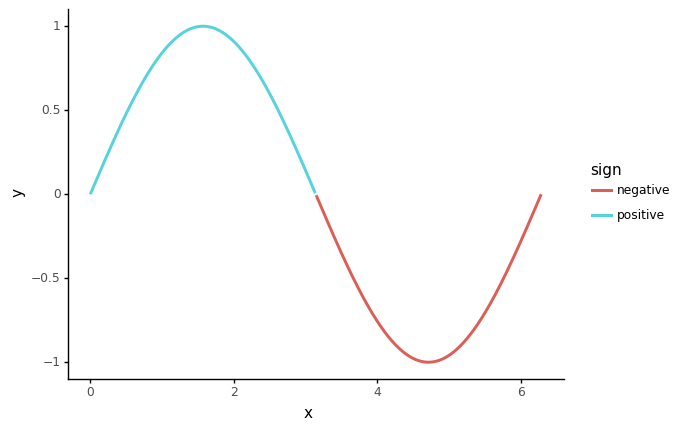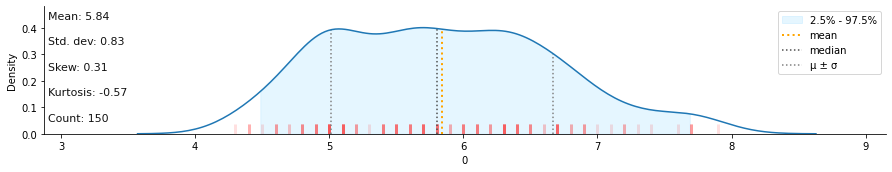# datar

A Grammar of Data Manipulation in python`datar` is a re-imagining of APIs of data manipulation libraries in python (currently only `pandas` supported) so that you can manipulate your data with it like with `dplyr` in `R`.

`datar` is an in-depth port of `tidyverse` packages, such as `dplyr`, `tidyr`, `forcats` and `tibble`, as well as some functions from base R.

## Installation

``````pip install -U datar

# install pdtypes support
pip install -U datar[pdtypes]

# install dependencies for modin as backend
pip install -U datar[modin]
# you may also need to install dependencies for modin engines
# pip install -U modin[ray]
``````

## Example usage

``````from datar import f
from datar.dplyr import mutate, filter, if_else
from datar.tibble import tibble
# or
# from datar.all import f, mutate, filter, if_else, tibble

df = tibble(
x=range(4),  # or f[:4]
y=['zero', 'one', 'two', 'three']
)
df >> mutate(z=f.x)
"""# output
x        y       z
<int64> <object> <int64>
0       0     zero       0
1       1      one       1
2       2      two       2
3       3    three       3
"""

df >> mutate(z=if_else(f.x>1, 1, 0))
"""# output:
x        y       z
<int64> <object> <int64>
0       0     zero       0
1       1      one       0
2       2      two       1
3       3    three       1
"""

df >> filter(f.x>1)
"""# output:
x        y
<int64> <object>
0       2      two
1       3    three
"""

df >> mutate(z=if_else(f.x>1, 1, 0)) >> filter(f.z==1)
"""# output:
x        y       z
<int64> <object> <int64>
0       2      two       1
1       3    three       1
"""
``````
``````# works with plotnine
# example grabbed from https://github.com/has2k1/plydata
import numpy
from datar.base import sin, pi
from plotnine import ggplot, aes, geom_line, theme_classic

df = tibble(x=numpy.linspace(0, 2*pi, 500))
(df >>
mutate(y=sin(f.x), sign=if_else(f.y>=0, "positive", "negative")) >>
ggplot(aes(x='x', y='y')) +
theme_classic() +
geom_line(aes(color='sign'), size=1.2))
````````````# easy to integrate with other libraries
# for example: klib
import klib
from datar.core.factory import verb_factory
from datar.datasets import iris
from datar.dplyr import pull

dist_plot = verb_factory(func=klib.dist_plot)
iris >> pull(f.Sepal_Length) >> dist_plot()
``````